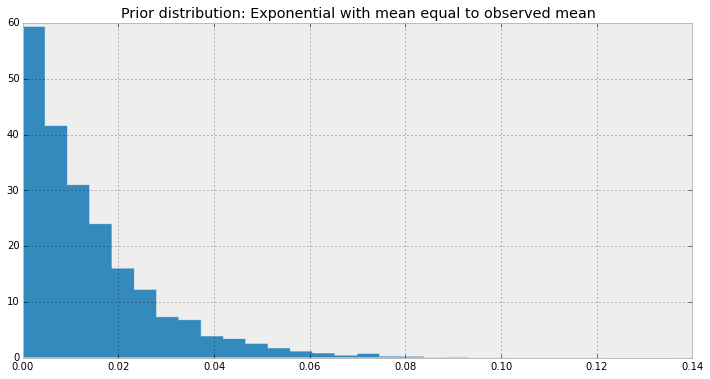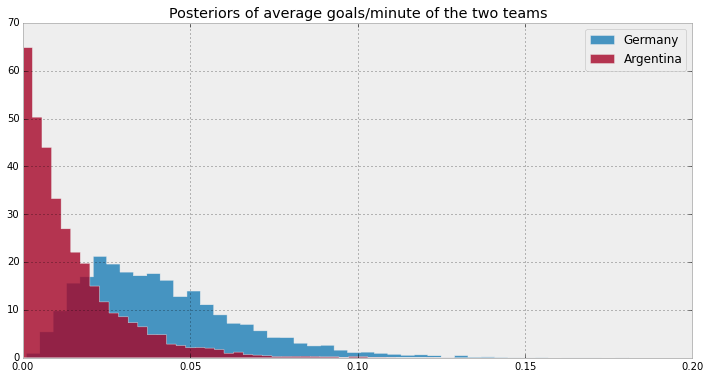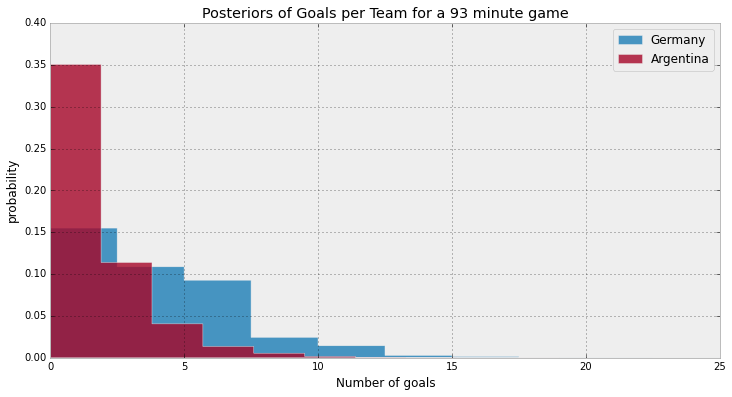### The World Cup: Germany vs. Argentina¶

This is a continuation of the Bayesian World Cup series: Allen Downey. has been posting neat little Bayesian problems and suggesting others replicating the solution in their frameworks of choice:

My other solution to the first problem is available here.

In [ ]:
import pymc as pm

%pylab inline
figsize(12,6)

In :
avg_goals_per_team = 1.34
duration_of_game = 93.

In :
german_prior = pm.Exponential('german_prior', duration_of_game/avg_goals_per_team)
arg_prior = pm.Exponential('arg_prior', duration_of_game/avg_goals_per_team)

sample = np.array([german_prior.random() for i in range(10000)])
hist(sample, bins=30, normed=True, histtype='stepfilled');
plt.title('Prior distribution: Exponential with mean equal to observed mean');In :
germany = pm.Poisson('germany_obs', german_prior, observed=True, value=)
argentina = pm.Poisson('arg_obs', arg_prior, observed=True, value=)

germany_predictive = pm.Poisson('germany_predictive', duration_of_game*german_prior)
arg_predictive = pm.Poisson('arg_predictive', duration_of_game*arg_prior)

In :
mcmc = pm.MCMC([germany, argentina, german_prior, arg_prior, germany_predictive, arg_predictive])

In :
mcmc.sample(20000, 5000)

 [-----------------100%-----------------] 20000 of 20000 complete in 5.5 sec
In :
german_lambda_trace = mcmc.trace('german_prior')[:]
arg_lambda_trace = mcmc.trace('arg_prior')[:]

In :
hist(german_lambda_trace, bins=45, histtype='stepfilled', label='Germany', alpha=0.9, normed=True);
hist(arg_lambda_trace, bins=45, histtype='stepfilled', label='Argentina', alpha=0.8, normed=True);
plt.legend();
plt.title('Posteriors of average goals/minute of the two teams');In :
german_post_trace = mcmc.trace('germany_predictive')[:]
arg_post_trace = mcmc.trace('arg_predictive')[:]

In :
hist(german_post_trace, bins=10, histtype='stepfilled', label='Germany', alpha=0.9, normed=True);
hist(arg_post_trace, bins=10, histtype='stepfilled', label='Argentina', alpha=0.8, normed=True);
plt.legend();
plt.title('Posteriors of Goals per Team for a 93 minute game');
plt.ylabel('probability')
plt.xlabel('Number of goals')

Out:
<matplotlib.text.Text at 0x117710450>In :
print "Probability of Germany winning: %.3f"%(german_post_trace > arg_post_trace).mean()
print "Probability of Argentina winning: %.3f"%(german_post_trace < arg_post_trace).mean()
print "Probability of tie: %.3f"%(german_post_trace == arg_post_trace).mean()

Probability of Germany winning: 0.728
Probability of Argentina winning: 0.159
Probability of tie: 0.113


Again, the differences between my solution and Allen's is due to the differences in our priors =)

In [ ]: